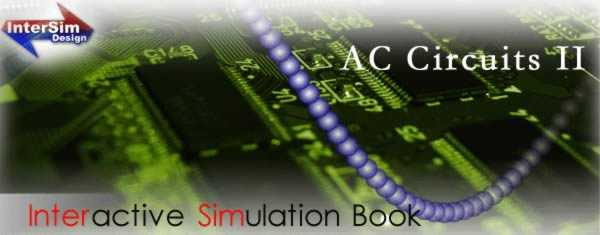Product Lineup

##### Chapter 1

You learn,

symbols you used in electric circuits,

various quantities which appear in electric circuits,

various formulas for electric engineering,

how to use Oscilloscope, and

how to use Frequency Analyzer.

### Chapter 2

You learn,

the relationships among the complex voltage ratio,

amplitude and phase characteristic.

how to calculate the complex voltage ratio, amplitude

characteristic and cut-off frequency response of a simple filter circuit,

frequency responses of a simple filter circuit, and

how to measure frequency response with the

frequency analyzer.

### Chapter 3

You learn,

resonance and how to calculate the characteristic values

of resonance,

how to measure the frequency responses of impedance and

the behavior of circuits in a resonant condition.

### Chapter 4

You learn,

how to calculate various types of powers in circuits.

how to calculate the power factor, and

how to measure the power and the power factor with

the oscilloscope.

### Lab

You learn,

how to design a circuit with the tools in a circuit

simulator, and

how to simulate a circuit that you designed with

measuring instruments in a circuit simulator.